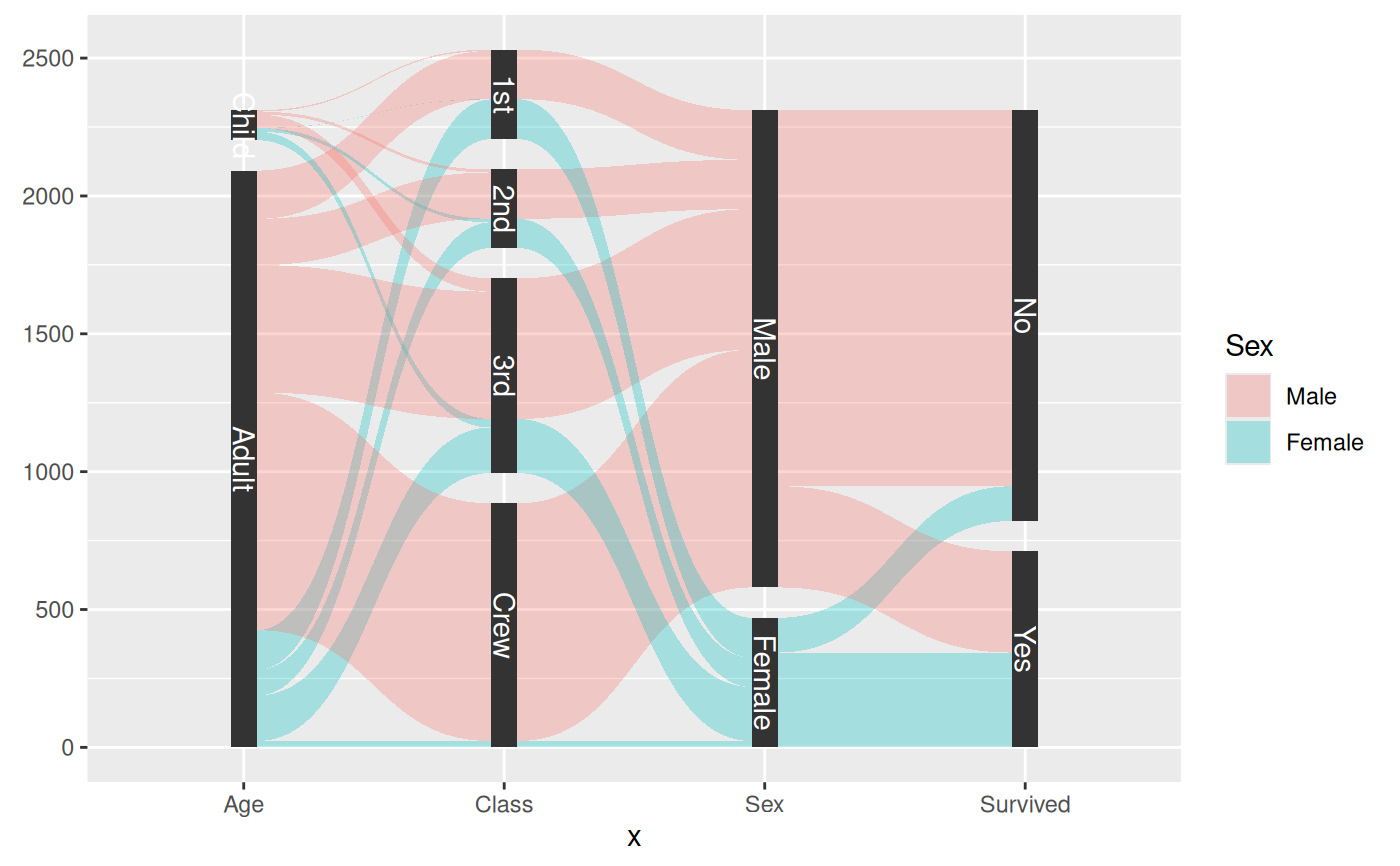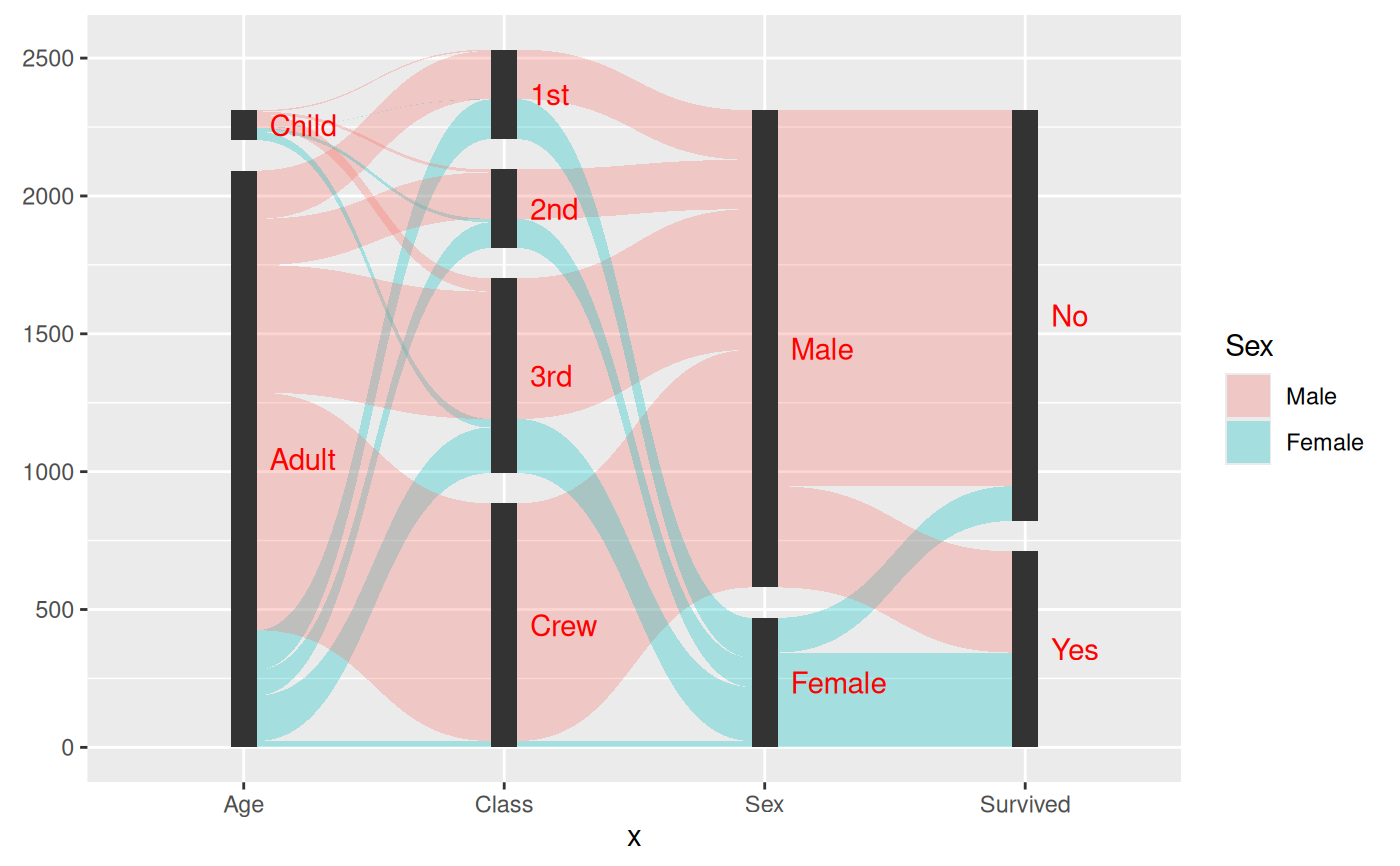A parallel sets diagram is a type of visualisation showing the interaction between multiple categorical variables. If the variables has an intrinsic order the representation can be thought of as a Sankey Diagram. If each variable is a point in time it will resemble an alluvial diagram.

stat_parallel_sets(
mapping = NULL,
data = NULL,
geom = "shape",
position = "identity",
n = 100,
strength = 0.5,
sep = 0.05,
axis.width = 0,
na.rm = FALSE,
orientation = NA,
show.legend = NA,
inherit.aes = TRUE,
...
)

geom_parallel_sets(
mapping = NULL,
data = NULL,
stat = "parallel_sets",
position = "identity",
n = 100,
na.rm = FALSE,
orientation = NA,
sep = 0.05,
strength = 0.5,
axis.width = 0,
show.legend = NA,
inherit.aes = TRUE,
...
)

stat_parallel_sets_axes(
mapping = NULL,
data = NULL,
geom = "parallel_sets_axes",
position = "identity",
sep = 0.05,
axis.width = 0,
na.rm = FALSE,
orientation = NA,
show.legend = NA,
inherit.aes = TRUE,
...
)

geom_parallel_sets_axes(
mapping = NULL,
data = NULL,
stat = "parallel_sets_axes",
position = "identity",
na.rm = FALSE,
orientation = NA,
show.legend = NA,
inherit.aes = TRUE,
...
)

geom_parallel_sets_labels(
mapping = NULL,
data = NULL,
stat = "parallel_sets_axes",
angle = -90,
nudge_x = 0,
nudge_y = 0,
position = "identity",
na.rm = FALSE,
orientation = NA,
show.legend = NA,
inherit.aes = TRUE,
...
)

## Arguments

mapping

Set of aesthetic mappings created by aes(). If specified and inherit.aes = TRUE (the default), it is combined with the default mapping at the top level of the plot. You must supply mapping if there is no plot mapping.

data

The data to be displayed in this layer. There are three options:

If NULL, the default, the data is inherited from the plot data as specified in the call to ggplot().

A data.frame, or other object, will override the plot data. All objects will be fortified to produce a data frame. See fortify() for which variables will be created.

A function will be called with a single argument, the plot data. The return value must be a data.frame, and will be used as the layer data. A function can be created from a formula (e.g. ~ head(.x, 10)).

geom

The geometric object to use to display the data, either as a ggproto Geom subclass or as a string naming the geom stripped of the geom_ prefix (e.g. "point" rather than "geom_point")

position

Position adjustment, either as a string naming the adjustment (e.g. "jitter" to use position_jitter), or the result of a call to a position adjustment function. Use the latter if you need to change the settings of the adjustment.

n

The number of points to create for each of the bounding diagonals

strength

The proportion to move the control point along the x-axis towards the other end of the bezier curve

sep

The proportional separation between categories within a variable

axis.width

The width of the area around each variable axis

na.rm

If FALSE, the default, missing values are removed with a warning. If TRUE, missing values are silently removed.

orientation

The orientation of the layer. The default (NA) automatically determines the orientation from the aesthetic mapping. In the rare event that this fails it can be given explicitly by setting orientation to either "x" or "y". See the Orientation section for more detail.

show.legend

logical. Should this layer be included in the legends? NA, the default, includes if any aesthetics are mapped. FALSE never includes, and TRUE always includes. It can also be a named logical vector to finely select the aesthetics to display.

inherit.aes

If FALSE, overrides the default aesthetics, rather than combining with them. This is most useful for helper functions that define both data and aesthetics and shouldn't inherit behaviour from the default plot specification, e.g. borders().

...

Other arguments passed on to layer(). These are often aesthetics, used to set an aesthetic to a fixed value, like colour = "red" or size = 3. They may also be parameters to the paired geom/stat.

stat

The statistical transformation to use on the data for this layer, either as a ggproto Geom subclass or as a string naming the stat stripped of the stat_ prefix (e.g. "count" rather than "stat_count")

angle

The angle of the axis label text

nudge_x, nudge_y

Horizontal and vertical adjustment to nudge labels by. Useful for offsetting text from the category segments.

## Details

In a parallel sets visualization each categorical variable will be assigned a position on the x-axis. The size of the intersection of categories from neighboring variables are then shown as thick diagonals, scaled by the sum of elements shared between the two categories. The natural data representation for such as plot is to have each categorical variable in a separate column and then have a column giving the amount/magnitude of the combination of levels in the row. This representation is unfortunately not fitting for the ggplot2 API which needs every position encoding in the same column. To make it easier to work with ggforce provides a helper gather_set_data(), which takes care of the transformation.

## Aesthetics

geom_parallel_sets understand the following aesthetics (required aesthetics are in bold):

• x|y

• id

• split

• value

• color

• fill

• size

• linetype

• alpha

• lineend

## Orientation

This geom treats each axis differently and, thus, can thus have two orientations. Often the orientation is easy to deduce from a combination of the given mappings and the types of positional scales in use. Thus, ggplot2 will by default try to guess which orientation the layer should have. Under rare circumstances, the orientation is ambiguous and guessing may fail. In that case the orientation can be specified directly using the orientation parameter, which can be either "x" or "y". The value gives the axis that the geom should run along, "x" being the default orientation you would expect for the geom.

## Author

Thomas Lin Pedersen

## Examples

data <- reshape2::melt(Titanic)
data <- gather_set_data(data, 1:4)

ggplot(data, aes(x, id = id, split = y, value = value)) +
geom_parallel_sets(aes(fill = Sex), alpha = 0.3, axis.width = 0.1) +
geom_parallel_sets_axes(axis.width = 0.1) +
geom_parallel_sets_labels(colour = 'white')# Use nudge_x to offset and hjust = 0 to left-justify label
ggplot(data, aes(x, id = id, split = y, value = value)) +
geom_parallel_sets(aes(fill = Sex), alpha = 0.3, axis.width = 0.1) +
geom_parallel_sets_axes(axis.width = 0.1) +
geom_parallel_sets_labels(colour = 'red', angle = 0, nudge_x = 0.1, hjust = 0)